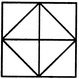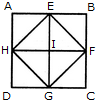# Non Verbal Reasoning - Analytical Reasoning - Discussion

Discussion Forum : Analytical Reasoning - Section 1 (Q.No. 19)
19.

Find the number of triangles in the given figure.8
10
12
14
Explanation:

The figure may be labelled as shown.The simplest triangles are AEH, EHI, EBF, EFI, FGC, IFG, DGH and HIG i.e. 8 in number.

The triangles composed of two components each are HEF, EFG, HFG and EFG i.e. 4 in number.

Thus, there are 8 + 4 = 12 triangles in the figure.

Discussion:
2 comments Page 1 of 1.

Deepanraj said:   6 years ago
I think, the answer is 14.

Riya said:   7 years ago
Last is wrong EFG and right answer is EHG.